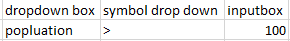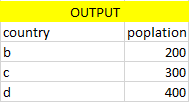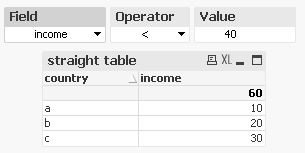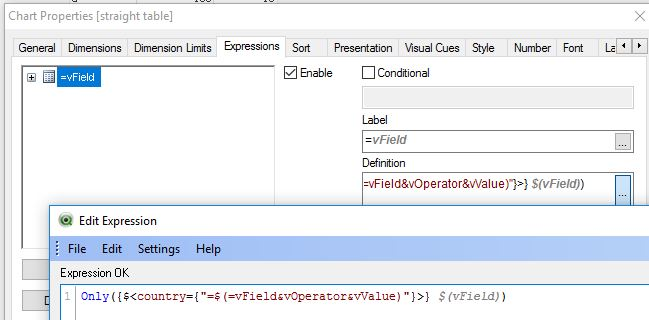# New to QlikView

Discussion board where members can get started with QlikView.

HighlightedNot applicable

## calculating values using >,< symbols and inputbox

Hi All
I have data as below

country    Population   income
a           100          10
b           200          20
c           300          30
d           400          40

i need  drop down list box, in which i want population or income as field values
i need another drop down  list box  which should contain >,<,>=,<= as values
i need a input box where i can give input values as numbers

my selection will be as belowmy output will be

straight table3 RepliesMVP

## Re: calculating values using >,< symbols and inputbox

Create two Variables.

Symbol and Value

SUM({<country = {"=Sum(Population)\$(Symbol)\$(Value)"}>}Population)MVP

## Re: calculating values using >,< symbols and inputbox

If you want to load Symbol as Inline table and use them as List box Selection... add below in your script..

Load * Inline

[

Symbol

<

<=

>

>=

];

Now use expression as below.

SUM({<country = {"=Sum(Population)\$(=GetFieldSelections(Symbol))\$(Value)"}>}Population)MVP

## Re: calculating values using >,< symbols and inputbox

Hi,

some similar solution might be:```Only({\$<country={"=\$(=vField&vOperator&vValue)"}>} \$(vField))
```

see also:

The Magic of Variables

The Little Equals Sign

The Magic of Dollar Expansions

hope this helps

regards

Marco